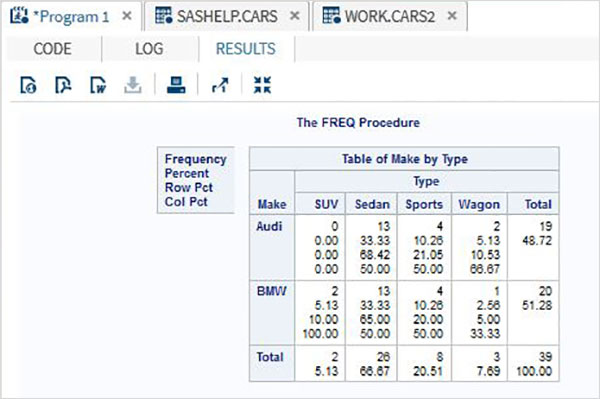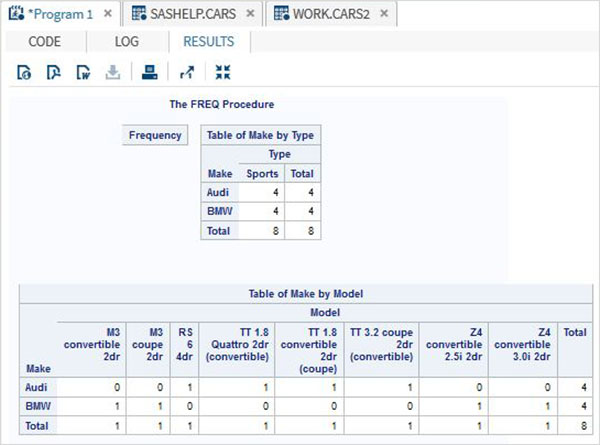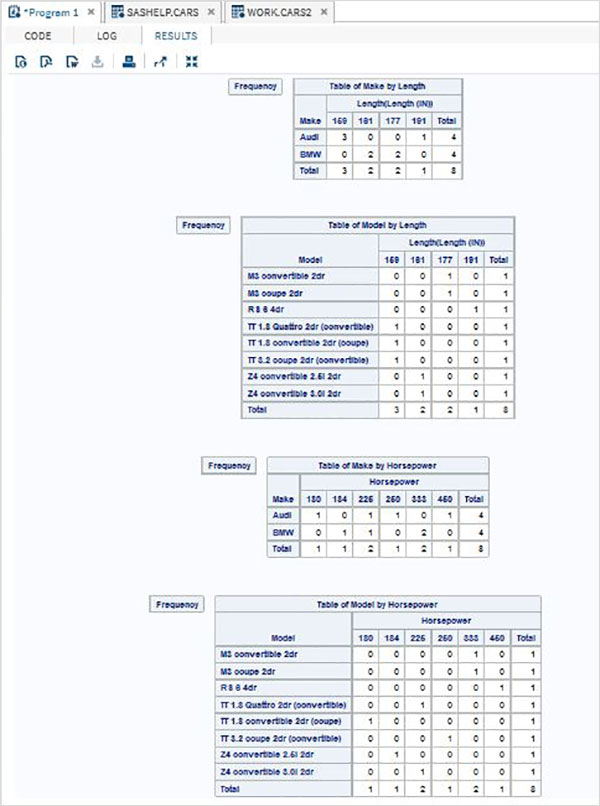# SAS - Cross Tabulations

Cross tabulation involves producing cross tables also called contingent tables using all possible combinations of two or more variables. In SAS it is created using PROC FREQ along with the TABLES option. For example - if we need the frequency of each model for each make in each car type category, then we need to use the TABLES option of PROC FREQ.

## Syntax

The basic syntax for applying cross tabulation in SAS is −

```PROC FREQ DATA = dataset;
TABLES variable_1*Variable_2;
```

Following is the description of the parameters used −

• Dataset is the name of the dataset.

• Variable_1 and Variable_2 are the variable names of the dataset whose frequency distribution needs to be calculated.

### Example

Consider the case of finding how many car types are available under each car brand from the dataset cars1 which is created form SASHELP.CARS as shown below. In this case we need the individual frequency values as well as the sum of the frequency values across the makes and across the types. We can observer that the result shows values across the rows and the columns.

```PROC SQL;
create table CARS1 as
SELECT make, type, invoice, horsepower, length, weight
FROM
SASHELP.CARS
WHERE make in ('Audi','BMW')
;
RUN;

proc FREQ data = CARS1;
tables make*type;
run;
```

When the above code is executed, we get the following result −## Cross tabulation of 3 Variables

When we have three variables we can group 2 of them and cross tabulate each of these two with the third varaible. So in the result we have two cross tables.

### Example

In the below example we find the frequency of each type of car and each model of car with respect to the make of the car. Also we use the nocol and norow option to avoid the sum and percentage values.

```proc FREQ data = CARS2 ;
tables make * (type model)  / nocol norow nopercent;
run;
```

When the above code is executed, we get the following result −## Cross tabulation of 4 Variables

With 4 variables, the number of paired combinations increases to 4. Each variable from group 1 is paired with each variable of group 2.

### Example

In the below example we find the frequency of length of the car for each make and each model. Similarly the frequency of horsepower for each make and each model.

```proc FREQ data = CARS2 ;
tables (make model) * (length  horsepower)  / nocol norow nopercent;
run;
```

When the above code is executed, we get the following result −